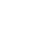# Checking simple equations or inequalities with z3

When programming, you sometimes need to make sure that a given formula is correct. Of course, you can rely on your mastery of high-school mathematics, but human beings, in general, are terrible at formal mathematics.

Thankfully, you can outsource simple problems to a software library. If you are a Python user, you can install z3 relatively with pip: pip install z3-solver. You are then good to go!

Suppose that you want to be sure that ( 1 + y ) / 2 < y for all 32-bit integers y. You can check it with the following Python script:

```import z3
y = z3.BitVec("y", 32)
s = z3.Solver()
s.add( ( 1 + y ) / 2 >= y )
if(s.check() == z3.sat):
model = s.model()
print(model)
```

We construct a “bit vector” spanning 32 bits to represent our integer. The z3 library considers such a number by default as a signed integer going from -2147483648 to 2147483647.

We check the reverse inequality, because we are looking for a counterexample. When no counterexample can be found, we know that our inequality holds.

Running the above script, Python prints back the integer 2863038463. How can this be given that we said that z3 assumed that we were using numbers from -2147483648 to 2147483647?  The z3 library always prints out a positive integer, and it is our job to reinterpret it: the number 2147483647 is fine, the number 2147483648 becomes -2147483648, the number 2147483649 becomes -2147483647 and so forth. This representation which “wraps around” is called two’s complement. So 2863038463 is actually interpreted as a negative value. The exact value is not too important: what matters is that our inequality (( 1 + y ) / 2 < y) is false when y is negative. It is clear enough: setting the variable to -1, I get 0 < -1.  For zero, the inequality is also false. Let me add as a condition that the variable is positive:

```import z3
y = z3.BitVec("y", 32)
s = z3.Solver()
s.add( ( 1 + y ) / 2 >= y )

if(s.check() == z3.sat):
model = s.model()
print(model)
```

This time, I get 1 as a possible solution. Damn it! Let us exclude it as well:

```import z3
y = z3.BitVec("y", 32)
s = z3.Solver()
s.add( ( 1 + y ) / 2 >= y )

if(s.check() == z3.sat):
model = s.model()
print(model)
```

Given this last script nothing is printed out which proves that my inequality is valid, as long as the variable is greater than 1.

Given that we have showed that ( 1 + y ) / 2 < y, then maybe ( 1 + y )  < 2 * y is true as well?

```import z3
y = z3.BitVec("y", 32)
s = z3.Solver()
s.add( ( 1 + y ) >= 2 * y )

if(s.check() == z3.sat):
model = s.model()
print(model)
```

This script returns 1412098654. Twice that value is 2824197308 which is interpreted as a negative number using two’s complement. To avoid the overflow, we can put another condition on the variable:

```import z3
y = z3.BitVec("y", 32)
s = z3.Solver()
s.add( ( 1 + y ) / 2 >= y )

if(s.check() == z3.sat):
model = s.model()
print(model)

```

This time the result holds!

As you can see, it is quite a lot of work and it unlikely that anyone would be able to fully test out a non-trivial program in this manner.### Daniel Lemire

A computer science professor at the University of Quebec (TELUQ).

## 2 thoughts on “Checking simple equations or inequalities with z3”

1.Antosha says:

// the number 2147483648 becomes -1 //
It becomes -2147483648.

1.Daniel Lemire says:

You are absolutely correct. Thank you for catching and reporting this mistake.

You may subscribe to this blog by email.